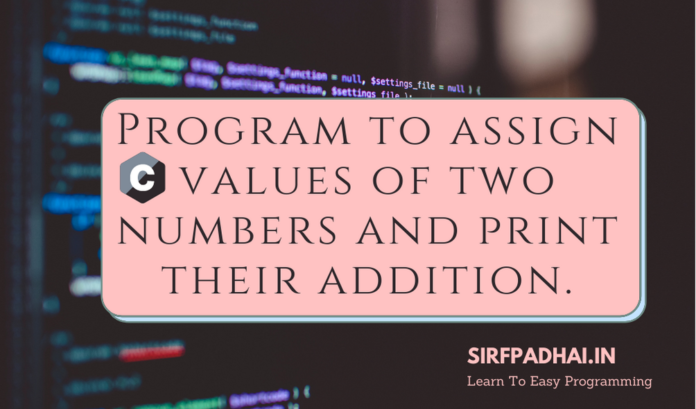# Program to assign values of two numbers and print their addition.In this program we will learn that how we can add two digits using C programming, for this we will first get the user to input two numbers and then add them using + operator.

### 1.Program to assign values of two numbers and print their addition?

Program

``````#include<stdio.h>

#include<conio.h>

void main()

{

int a=10,b=20;

clrscr();       //Devc++ compiler does not use clrscr.

int ans = a + b;

getch();

}``````

Output

``Addition is : 30``

### 2.Program to accept values of two numbers and print their addition.

Program

``````#include<stdio.h>

#include<conio.h>

void main()

{

int a,b,ans;

clrscr();         //Devc++ compiler does not use clrscr.

printf("Enter 1st number:");

scanf("%d",&a);

printf("Enter 2nd number:");

scanf("%d",&b);

ans = a + b;

getch();

}``````

Output

``````Enter 1st number:52
Enter 2nd number:79